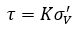The Rocscience International Conference 2021 Proceedings are now available. Read Now

# Vertical Stress Ratio

With the Vertical Stress Ratio model, the shear strength at the base of each column is determined by multiplying the effective vertical (overburden) stress by a constant K for the material.## Vertical Stress Ratio

The "vertical stress ratio" K is simply a constant, equal to the ratio of the shear strength to the vertical stress. (e.g. if K = 0.3, then the shear strength will be 30 % of the effective vertical stress.)

The effective vertical stress is computed from the total weight of each column, and the pore pressure acting at the center of the base of each column.

## Minimum Shear Strength

The Minimum Shear Strength option allows you to define a minimum value of shear strength for the Vertical Stress Ratio option.

## Maximum Shear Strength

The Maximum Shear Strength option allows you to define a maximum value of shear strength for the Vertical Stress Ratio option.

## Tensile Strength

See the Tensile Strength topic.